# Plotting Histogram in Python using Matplotlib

Histograms are a fundamental tool in data visualization, providing a graphical representation of the distribution of data. They are particularly useful for exploring continuous data, such as numerical measurements or sensor readings. This article will guide you through the process of Plot Histogram in Python using Matplotlib, covering the essential steps from data preparation to generating the histogram plot.

### What is Matplotlib Histograms?

A Histogram represents data provided in the form of some groups. It is an accurate method for the graphical representation of numerical data distribution. It is a type of bar plot where the X-axis represents the bin ranges while the Y-axis gives information about frequency.

## Creating a Matplotlib Histogram

To create a Matplotlib histogram the first step is to create a bin of the ranges, then distribute the whole range of the values into a series of intervals, and count the values that fall into each of the intervals. Bins are clearly identified as consecutive, non-overlapping intervals of variables.The matplotlib.pyplot.hist() function is used to compute and create a histogram of x.

The following table shows the parameters accepted by matplotlib.pyplot.hist() function :

Attribute Parameter
x array or sequence of array
bins optional parameter contains integer or sequence or strings
density optional parameter contains boolean values
range optional parameter represents upper and lower range of bins
histtype optional parameter used to create type of histogram [bar, barstacked, step, stepfilled], default is “bar”
align optional parameter controls the plotting of histogram [left, right, mid]
weights optional parameter contains array of weights having same dimensions as x
bottom location of the baseline of each bin
rwidth optional parameter which is relative width of the bars with respect to bin width
color optional parameter used to set color or sequence of color specs
label optional parameter string or sequence of string to match with multiple datasets
log optional parameter used to set histogram axis on log scale

## Plotting Histogram in Python using Matplotlib

Here we will see different methods of Plotting Matplotlib Histograms in Python:

• Basic Histogram
• Customized Histogram with Density Plot
• Customized Histogram with Watermark
• Multiple Histograms with Subplots
• Stacked Histogram
• 2D Histogram (Hexbin Plot)

### Create a Basic Histogram

Let’s create a basic histogram using Matplotlib in Python of some random values.

## Python3

 `import` `matplotlib.pyplot as plt` `import` `numpy as np`   `# Generate random data for the histogram` `data ``=` `np.random.randn(``1000``)`   `# Plotting a basic histogram` `plt.hist(data, bins``=``30``, color``=``'skyblue'``, edgecolor``=``'black'``)`   `# Adding labels and title` `plt.xlabel(``'Values'``)` `plt.ylabel(``'Frequency'``)` `plt.title(``'Basic Histogram'``)`   `# Display the plot` `plt.show()`

Output: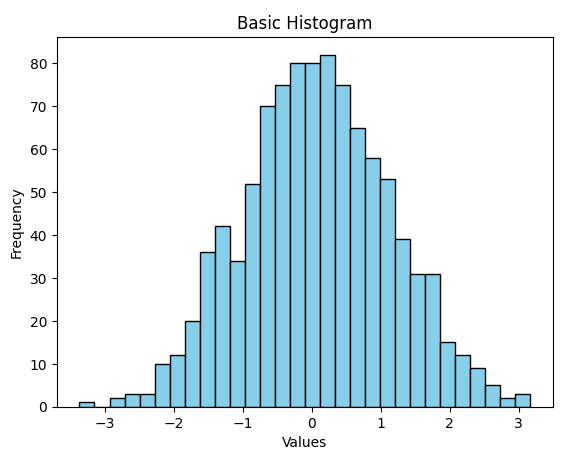### Customized Histogram with Density Plot

Let’s create a customized histogram with a density plot using Matplotlib and Seaborn in Python. The resulting plot visualizes the distribution of random data with a smooth density estimate.

## Python3

 `import` `matplotlib.pyplot as plt` `import` `seaborn as sns` `import` `numpy as np`   `# Generate random data for the histogram` `data ``=` `np.random.randn(``1000``)`   `# Creating a customized histogram with a density plot` `sns.histplot(data, bins``=``30``, kde``=``True``, color``=``'lightgreen'``, edgecolor``=``'red'``)`   `# Adding labels and title` `plt.xlabel(``'Values'``)` `plt.ylabel(``'Density'``)` `plt.title(``'Customized Histogram with Density Plot'``)`   `# Display the plot` `plt.show()`

Output: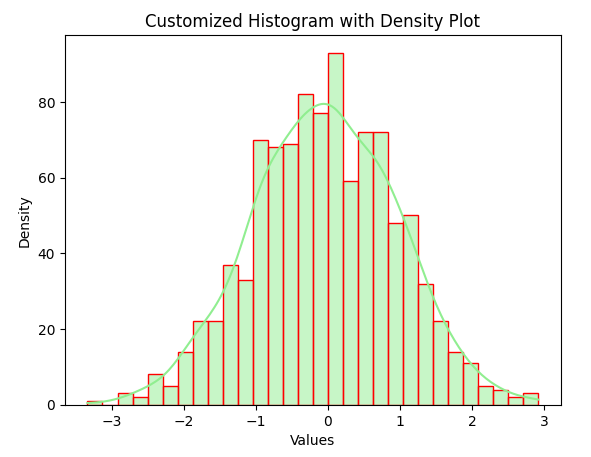### Customized Histogram with Watermark

Create a customized customized histogram using Matplotlib in Python with specific features. It includes additional styling elements, such as removing axis ticks, adding padding, and setting a color gradient for better visualization.

## Python3

 `import` `matplotlib.pyplot as plt` `import` `numpy as np` `from` `matplotlib ``import` `colors` `from` `matplotlib.ticker ``import` `PercentFormatter`     `# Creating dataset` `np.random.seed(``23685752``)` `N_points ``=` `10000` `n_bins ``=` `20`   `# Creating distribution` `x ``=` `np.random.randn(N_points)` `y ``=` `.``8` `*``*` `x ``+` `np.random.randn(``10000``) ``+` `25` `legend ``=` `[``'distribution'``]`   `# Creating histogram` `fig, axs ``=` `plt.subplots(``1``, ``1``,` `                        ``figsize ``=``(``10``, ``7``), ` `                        ``tight_layout ``=` `True``)`     `# Remove axes splines ` `for` `s ``in` `[``'top'``, ``'bottom'``, ``'left'``, ``'right'``]: ` `    ``axs.spines[s].set_visible(``False``) `   `# Remove x, y ticks` `axs.xaxis.set_ticks_position(``'none'``) ` `axs.yaxis.set_ticks_position(``'none'``) ` `  `  `# Add padding between axes and labels ` `axs.xaxis.set_tick_params(pad ``=` `5``) ` `axs.yaxis.set_tick_params(pad ``=` `10``) `   `# Add x, y gridlines ` `axs.grid(b ``=` `True``, color ``=``'grey'``, ` `        ``linestyle ``=``'-.'``, linewidth ``=` `0.5``, ` `        ``alpha ``=` `0.6``) `   `# Add Text watermark ` `fig.text(``0.9``, ``0.15``, ``'Jeeteshgavande30'``, ` `         ``fontsize ``=` `12``, ` `         ``color ``=``'red'``,` `         ``ha ``=``'right'``,` `         ``va ``=``'bottom'``, ` `         ``alpha ``=` `0.7``) `   `# Creating histogram` `N, bins, patches ``=` `axs.hist(x, bins ``=` `n_bins)`   `# Setting color` `fracs ``=` `((N``*``*``(``1` `/` `5``)) ``/` `N.``max``())` `norm ``=` `colors.Normalize(fracs.``min``(), fracs.``max``())`   `for` `thisfrac, thispatch ``in` `zip``(fracs, patches):` `    ``color ``=` `plt.cm.viridis(norm(thisfrac))` `    ``thispatch.set_facecolor(color)`   `# Adding extra features    ` `plt.xlabel(``"X-axis"``)` `plt.ylabel(``"y-axis"``)` `plt.legend(legend)` `plt.title(``'Customized histogram'``)`   `# Show plot` `plt.show()`

Output :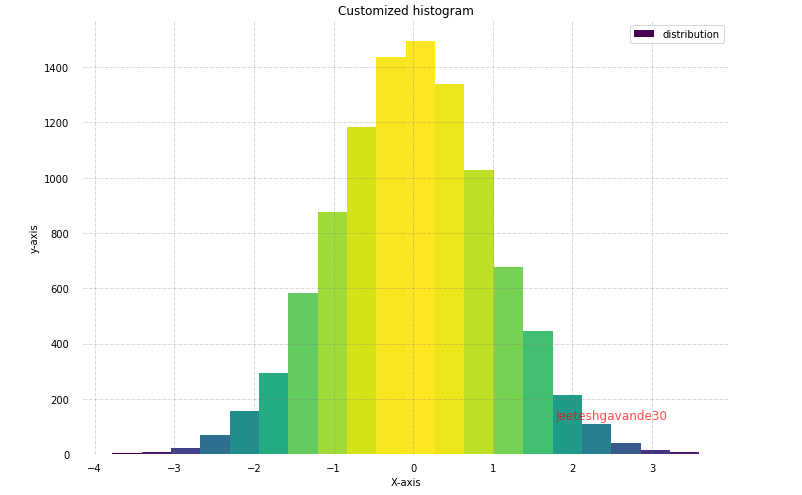### Multiple Histograms with Subplots

Let’s generates two histograms side by side using Matplotlib in Python, each with its own set of random data and provides a visual comparison of the distributions of `data1` and `data2` using histograms.

## Python3

 `import` `matplotlib.pyplot as plt` `import` `numpy as np`   `# Generate random data for multiple histograms` `data1 ``=` `np.random.randn(``1000``)` `data2 ``=` `np.random.normal(loc``=``3``, scale``=``1``, size``=``1000``)`   `# Creating subplots with multiple histograms` `fig, axes ``=` `plt.subplots(nrows``=``1``, ncols``=``2``, figsize``=``(``12``, ``4``))`   `axes[``0``].hist(data1, bins``=``30``, color``=``'Yellow'``, edgecolor``=``'black'``)` `axes[``0``].set_title(``'Histogram 1'``)`   `axes[``1``].hist(data2, bins``=``30``, color``=``'Pink'``, edgecolor``=``'black'``)` `axes[``1``].set_title(``'Histogram 2'``)`   `# Adding labels and title` `for` `ax ``in` `axes:` `    ``ax.set_xlabel(``'Values'``)` `    ``ax.set_ylabel(``'Frequency'``)`   `# Adjusting layout for better spacing` `plt.tight_layout()`   `# Display the figure` `plt.show()`

Output: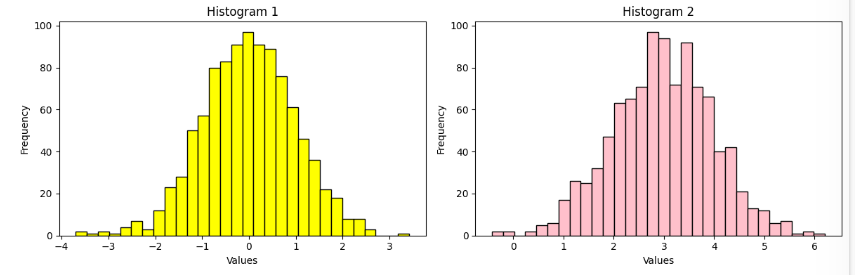### Stacked Histogram using Matplotlib

Let’s generates a stacked histogram using Matplotlib in Python, representing two datasets with different random data distributions. The stacked histogram provides insights into the combined frequency distribution of the two datasets.

## Python3

 `import` `matplotlib.pyplot as plt` `import` `numpy as np`   `# Generate random data for stacked histograms` `data1 ``=` `np.random.randn(``1000``)` `data2 ``=` `np.random.normal(loc``=``3``, scale``=``1``, size``=``1000``)`   `# Creating a stacked histogram` `plt.hist([data1, data2], bins``=``30``, stacked``=``True``, color``=``[``'cyan'``, ``'Purple'``], edgecolor``=``'black'``)`   `# Adding labels and title` `plt.xlabel(``'Values'``)` `plt.ylabel(``'Frequency'``)` `plt.title(``'Stacked Histogram'``)`   `# Adding legend` `plt.legend([``'Dataset 1'``, ``'Dataset 2'``])`   `# Display the plot` `plt.show()`

Output: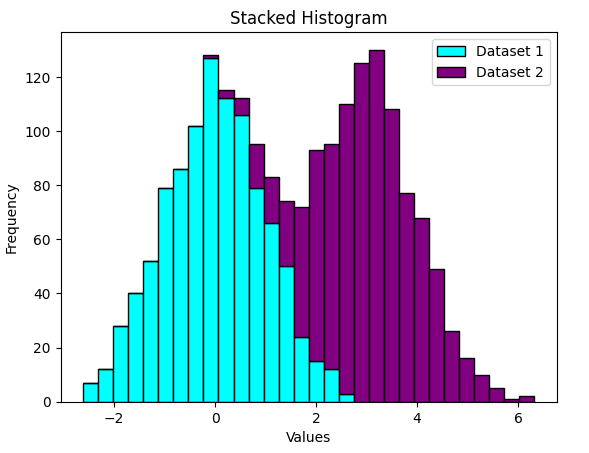### Plot 2D Histogram (Hexbin Plot) using Matplotlib

Let’s generates a 2D hexbin plot using Matplotlib in Python, provides a visual representation of the 2D data distribution, where hexagons convey the density of data points. The colorbar helps interpret the density of points in different regions of the plot.

## Python3

 `import` `matplotlib.pyplot as plt` `import` `numpy as np`   `# Generate random 2D data for hexbin plot` `x ``=` `np.random.randn(``1000``)` `y ``=` `2` `*` `x ``+` `np.random.normal(size``=``1000``)`   `# Creating a 2D histogram (hexbin plot)` `plt.hexbin(x, y, gridsize``=``30``, cmap``=``'Blues'``)`   `# Adding labels and title` `plt.xlabel(``'X values'``)` `plt.ylabel(``'Y values'``)` `plt.title(``'2D Histogram (Hexbin Plot)'``)`   `# Adding colorbar` `plt.colorbar()`   `# Display the plot` `plt.show()`

Output: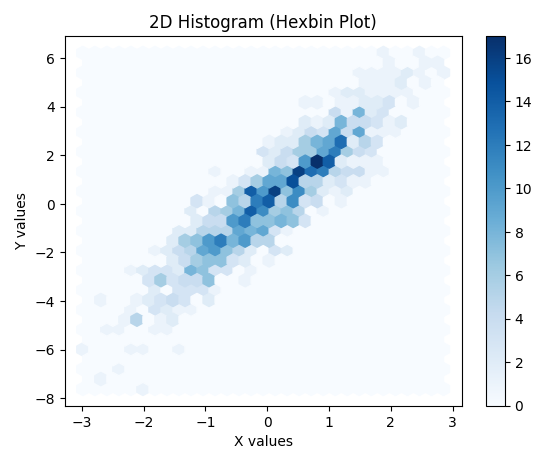## Conclusion

Plotting Matplotlib histograms is a simple and straightforward process. By using the `hist()` function, we can easily create histograms with different bin widths and bin edges. We can also customize the appearance of histograms to meet our needs

Whether you're preparing for your first job interview or aiming to upskill in this ever-evolving tech landscape, GeeksforGeeks Courses are your key to success. We provide top-quality content at affordable prices, all geared towards accelerating your growth in a time-bound manner. Join the millions we've already empowered, and we're here to do the same for you. Don't miss out - check it out now!

Previous
Next